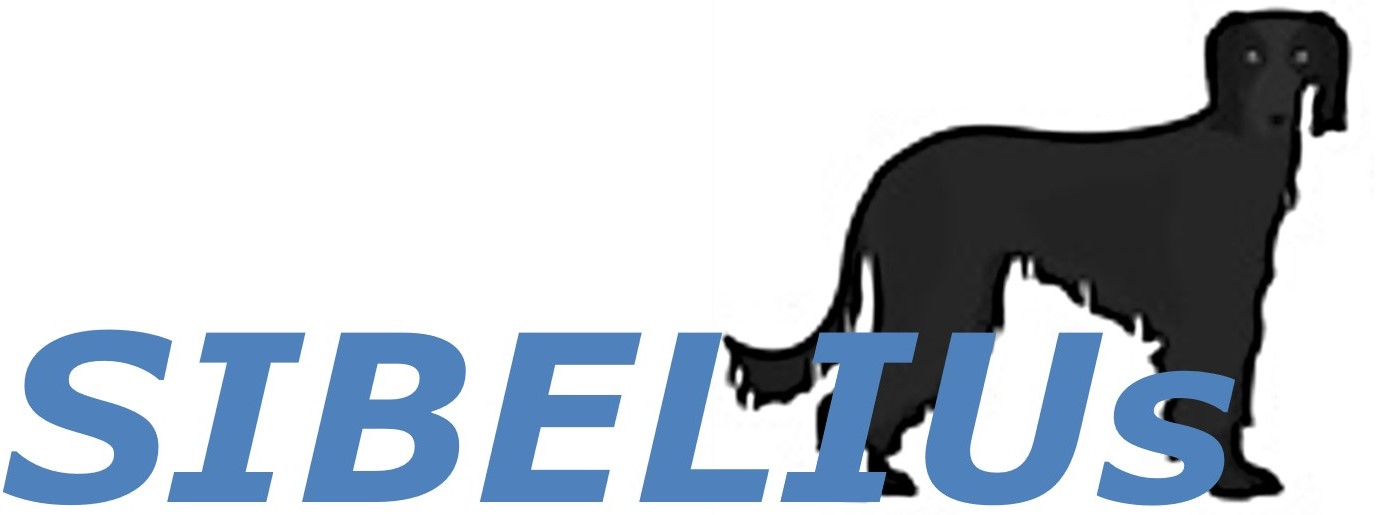# Exercises¶

This notebook aims to provide a list of exercises useful to start practising your programming skills

## How to start¶

Opening a new notebook, you should start importing the modules that are needed for the notebook to work. For instance: import numpy, import matplotlib, import datacube, etc.

In the following cells there will be some exercise with an hidden solution so that you can try to solve the problem yourself. Click on the green button 'Show Solution' to compare your solution with the one provided.

Be aware: in programming there are multiple right solutions, so it is only important that you find the same results.

## Working with strings¶

### 1) Given the following strings: s1 = 'Hi there' and s2 = 'my name is Ernest'¶

    i) Create a single string s3, which is the union of s1 and s2
    ii) Split s3 creating a list of words, for example: ['Hi','there','my','name','is','Ernest']
    iii) Replace the name Ernest with Bob in s3
    iv) Remove the word 'there ' in s3

### 2) Given s1 = 'Hello world!' , s2 = 'one two three four five'¶

i) Find the lenght (number of characters) of s1 and s2
ii) Create a new string s3 that has the first two letters of s1 and the last two of s2
iii) Create a new string s4 that is the sum of s1 and s2 (leaving an empty space between s1 and s2), and count how many times the expression 'wo' appears
iv) add the expression 'bye bye' in the middle of s4